# TN Board SSLC Class 10 Maths Important Questions And Solutions

Class 10 Maths of TN board is a very important subject and an easy one from the point of view of scoring. But, instead of this, some students struggle to score good marks for the same subject. As Class 10 is a crucial part of any students life so it is necessary to tackle maths subject effectively. Class 10 board exam is an excellent litmus test which signifies the level of sincerity and sense of responsibility the student carriers. Hence, the students who wish to score high marks in the SSLC exams, will find this Tamil Nadu Board SSLC Class 10 Maths Important Questions with Solutions pretty useful to prepare for the exams.

## Tamil Nadu Board SSLC Class 10 Maths Important Questions and Solutions

TN board Class 10 important questions were prepared after a thorough analysis of previous year question paper and past TN board exam pattern. Students must practice these important questions of Class 10 maths so that they can tackle Class 10 Maths examination with confidence. By knowing the important questions and doing a regular practice of it students can score 100% marks. Download the pdf version of Class 10 maths important questions and try to solve every question.

These important questions are important to self-evaluate students preparation level. It helps them to cross check once whether they have covered the entire Tamilnadu Board Class 10 Maths syllabus or something they have missed it. These important questions prove to be a valuable aid to students while preparing for their Class 10 board exams. Effective practice is a must if you want to score good marks in your TN Board Class 10 Mathematics.

### Download TN Board Class 10 Maths Important Questions And Solutions PDF

Question 1: Let X = {1, 2, 3, 4}. Examine whether the relation f = {(2, 3), (1, 4), (2, 1), (3, 2), (4, 4)} is a function from X to X or not. Explain.

Solution:

X = {1, 2, 3, 4}

f = {(2, 3), (1, 4), (2, 1), (3, 2), (4, 4)}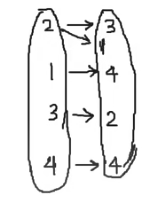2 has two output values which are 3 and 1.

So, f is not a function.

Question 2: Three numbers are in the ratio 2:5:7. If 7 is subtracted from the second, the resulting numbers form an arithmetic sequence. Determine the numbers.

Solution:

Let the three numbers be 2x, 5x and 7x.

It is given that the numbers 2x, 5x − 7, 7x are in an arithmetic progression.

So, 2 (5x − 7) = 2x + 7x

⇒10x − 14 = 9x

⇒ x = 14

2x = 2 * 14 = 28

5x = 5 * 14 = 70

7x = 7 * 14 = 98

Therefore the numbers are 28, 70, 98.

Question 3: The centre of a circle is at (− 6, 4). If one end of a diameter of the circle is at the origin, then find the other end.

Solution:

The one end of the diameter is at the origin, that is A (0, 0).

Let B (a, b) be another endpoint of the diameter & C (- 6, 4) is the centre of the circle. The centre is the midpoint of the circle.

Using the midpoint formula,

Midpoint = (x₁ + x₂) / 2 , (y₁ + y₂) / 2

x = – 6 , y = 4 , x1 = 0, y1 = 0, x2 = a, y2 = b

(-6 , 4) = (0 + a) / 2 , (0 + b) / 2

(-6 , 4) = a / 2 , b / 2

a / 2 = – 6

a = – 6 * 2

a = – 12

b / 2 = 4

b = 4 * 2

b = 8

Hence, the other endpoint of the diameter is (-12, 8).

Question 4: A ladder leaning against a vertical wall, makes an angle of 60° with the ground. The foot of the ladder 3.5m away from the wall. Find the length of the ladder.

Solution: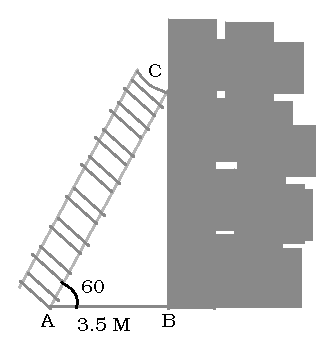Let AC be the length of the ladder, AB be the distance between the foot of the ladder and the base of the wall.

AB = 3.5 m

In right-angled ∆ABC,

cos 600 = AB / AC

1 / 2 = 3.5 / AC

AC = 3.5 × 2

AC = 7 m

The length of the ladder is 7m.

Question 5: A right circular cylinder has a radius of 14cm and a height of 8cm. Find its curved surface area.

Solution:

Let r be the radius and h be the height of the cylinder.

Curved surface area of right cylinder = 2πrh

Total surface area of right cylinder = 2πr (r + h)

It is given that radius = 14 cm and height = 8cm

Curved Surface Area = 2 × (22 / 7) × 14 × 8

= 704 cm²

Total surface area = 2 × (22 / 7) × 14 (14 + 8)

= 1936 cm²

Question 6: The circumference of the base of a 12m high wooden solid come is 44m. Find the volume.

Solution:

The circumference of the cone is 44m.

Height of the cone = 12m

Circumference of the cone is given by 2πr.

2πr = 44

2 × (22 / 7) × r = 44

r = 44 × (7 / 22) × 2

r = 7m

The volume of a cone is (1 / 3) πr2h.

= (1 / 3) × (22 / 7) × 7× 7 × 12

= 616 m3

Question 7: Calculate the standard deviation of the first 13 natural numbers.

Solution:

Standard deviation = √(n² -1) / 12

= √(13² -1) / 12

= √(169 -1) / 12

= √168 / 12

= √14

= 3.7416

= 3.74

Thus the standard deviation of the first 13 natural numbers is 3.74.

Question 8: Two coins are tossed together. What is the probability of getting at least one head?

Solution:

When two coins are tossed simultaneously, then the possibility of outcomes are: (HH), (HT), (TH), (TT)

Therefore, the total numbers of outcome are 22 = 4.

Let E be the event of getting at least 1 head.

E = {(HH , HT, TH}

n (E) = 3.

P(getting at least 1 head) = P (E) = n (E) / n (S) = 3 / 4

Question 9: Show that the lines 5x + 23y + 14 = 0 and 23x – 5y + 9 = 0 are perpendicular.

Solution:

5x + 23y + 14 = 0

23y = – 5x – 14

y = (-5x / 23) – (14 / 23)

m1 = (-5 / 23)

23x – 5y + 9 = 0

-5y = -23x – 9

y = (23 / 5) x + (9 / 5)

m2 = (23 / 5)

m1 m2 = (-5 / 23) * (23 / 5) = -1

m1 m2 = -1

The lines are perpendicular.

Question 10: Use Venn diagrams to verify De Morgan’s law for set difference A \ (B ∩ C) = (A \ B) ∪ (A \ C).

Solution: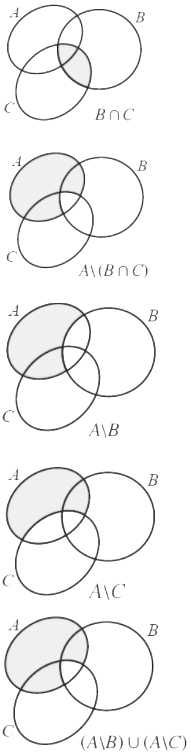Question 11: The speed of a boat in still water is 15 km/hr. It goes 30 km upstream and returns downstream to the original point in 4 hrs 30 minutes. Find the speed of the stream.

Solution:

Speed in still water = 15 km/hr

Total time taken = 4 hrs 30 min = 4 (1 / 2) hr = 9 / 2 hrs

Let x km/hr be the speed of the stream.

Speed of the boat during upstream = 15 – x

Speed of the boat during downstream = 15 + x

Time taken for upstream = T1 = 30 / (15 – x)

Time taken for downstream = T2 = 30 / (15 + x)

According to the given,

T1 + T2 = 9 / 2

[30 / (15 – x)] + [30 / (15 + x)] = 9 / 2

30 [(15 + x + 15 – x) / (15 – x) (15 + x)] = 9 / 2

30 / (225 – x2) = (9 / 2 × 30)

30 / (225 – x2) = 3 / 20

⇒ 600 = 675 – 3x2

⇒ 3x2 = 675 – 600

⇒ x2 = 75/3

⇒ x2 = 25

⇒ x = 5

Therefore, the speed of the stream = 5 km/hr.

Question 12: Draw the two tangents from a point which is 10cm away from the centre of a circle of radius 6cm. Also, measure the lengths of the tangents.

Solution:

Steps of construction:

[a] Draw a circle of radius 6cm with O as the centre.

[b] At a distance of 10 cm from O, mark a point P and join OP.

[c] A perpendicular bisector is drawn for OP and it meets OP at M.

[d] Another circle of radius MO is drawn with M as the centre.

[e] The two circles intersect at X and Y.

[f] PX and PY are joined.

They are required tangents.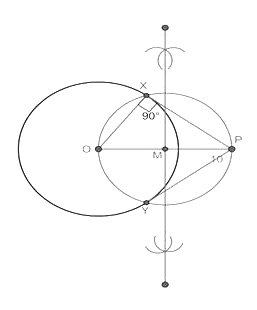Question 13: Construct a ∆PQR in which PQ = 5 cm, ∠P = 40°and the median PG from P to QR is 4.4 cm. Find the length of the altitude from P to QR.

Solution:

Steps of construction:

a. A line segment PQ = 5cm is drawn.

b. At P, draw PE and PF such that ∠QPE = 40° and ∠EPF = 90°.

c. A perpendicular bisector to PQ is drawn which meets PF at O and PQ at G.

c. A circle is drawn with O as centre and OP as the radius.

d. Arcs of 4.4 cm are marked on G which is on the circle of radius 4.4cm.

e. Join PR, RQ. ∆PQR is the required triangle.

f. Length of altitude is RM = 3 cm.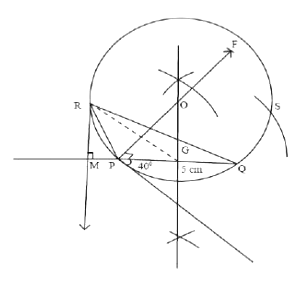Question 14: A boy is designing a diamond-shaped kite, as shown in the figure where AE = 16 cm, EC = 81 cm. He wants to use a straight crossbar BD. How long should it be?

Solution: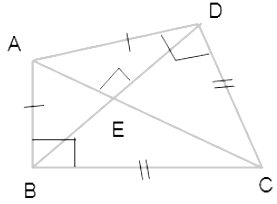Let ∠EAD = x —- (1)

⇒ ∠ADE = 90 – x (∵ AED = 90 and sum of angles of triangle is 180)

⇒ ∠EDC = x (∵ ∠ADC = 90o) —- (2)

⇒ ∠EAD = ∠EDC (by (1) and (2)) —- (3)

In ΔAED and ΔDEC,

∠AED = ∠DEC (both perpendicular to AC)

∴ ΔAED ∼ ΔDEC (∵ ΔAED ∼ ΔDEC)

EA / ED = ED / EC

⇒ 16 * 81 = ED2

⇒ ED = 36 cm

⇒ 2 * ED = DB = 72 cm

Question 15: A vertical tree is broken by the wind. The top of the tree touches the ground and makes an angle 30° with it. If the top of the tree touches the ground 30m away from its foot, then find the actual height of the tree.

Solution:

Let

OA be the tree with O as foot and A as the top.

B be the point of the break, such that OB = x and AB = y.

Height of tree = OA = x + y

In right-angled triangle ∆OAB,

OA = 30 m

OB = x m

AB = y m

=> tan 30° = OB / OA

=> tan 30° = x / 30

=> 1 / √3 = x / 30

=> (1 / √3) × 30 = x

=> x = 30 / √3

=> x = 10√3

=> cos 30° = OA / AB

=> cos 30° = 30 / y

=> √3 / 2 = 30 / y

=> y = 30 × (2 / √3)

=> y = 20√3

Actual height of the tree OA = OB + AB = x + y

= 10√3 + 20√3

= 30√3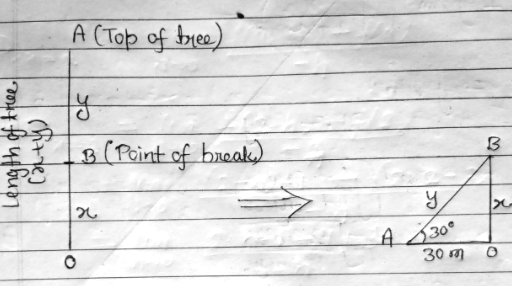Question 16: A cylindrical jar of diameter 14cm and depth 20cm is half-full of water. 300 lead shots of the same size are dropped into the jar and the level of water rises by 2.8 cm. Find the diameter of each lead shot.

Solution:

Diameter of cylindrical jar = D = 14 cm

Depth of the jar = H = 20 cm

Initial water level W1 = half of the jar = 10 cm

Then the water level was raised by 2.8 cm.

Number of lead shots = 300

Let the diameter of the lead shots be d cm.

The volume of the water in the jar before dropping the shots is given by

V = (π / 4) × D² × h

V1 = (3.14 / 4) × 14² × 10

V1 = 1538.6 cm³ ….. initial volume of water …. (1)

After dropping the 300 shots, water height increased by 2.8 cm.

New water level W2 = 10 + 2.8 = 12.8 cm

Total volume occupied by the water and the shots are given by

V2 = (π / 4) × D² × W2

V2 = (3.14 / 4) × 14² × 12.8

V2 = 1969.408 cm³ ……. (2)

The new water level volume is a combination of the volume of water and the volume of the lead shots.

If the volume of the single lead shot = Vs

V2 = V1 + 300Vs

300Vs = V2 – V1

300Vs = 1969.408 – 1538.6

300Vs = 430.808 cm³

∴ Vs = 1.436 cm³ …… (3) … Volume of a single shot

Vs = (4 / 3) ×π × r³ ….

1.436 = (4 / 3) × 3.14 × (d / 2)³

(d / 2)³ = (3 × 1.436) ÷ (4 × 3.14 )

(d / 2)³ = 0.343

d / 2 = ∛0.343

d / 2 = 0.7

d = 1.4 cm

Question 17: Find the area of the quadrilateral formed by the points (− 4, − 2), (− 3, − 5), (3, − 2) and (2, 3).

Solution: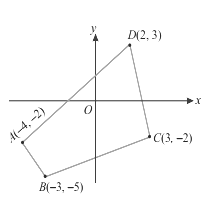The vertices are A (−4, −2), B (−3, −5), C (3, −2) and D (2, 3).

Area of the quadrilateral ABCD = (1 / 2) [(x1y2 + x2y3 + x3y1 + x4y1) – (x2y1 + x3y2 + x1y3 + x1y4]

= (1 / 2) [(20 + 6 + 9 − 4) − (6 − 15 − 4 − 12)]

= (1 / 2) (31 + 25)

= 28 square units

Question 18: Using clay, a student made a right circular cone of height 48cm and base radius 12cm. Another student reshapes it in the form of a sphere. Find the radius of the sphere.

Solution:

Height of cone (h) = 48 cm

Base radius (r) = 12 cm

Volume of cone = (1 / 3) 𝛑r2h

= (1 / 3) 𝛑 12 * 12 * 48

= 2304𝛑

Volume of sphere = (4 / 3) 𝛑r3

(4 / 3) 𝛑r3 = 2304

⇒ r3 = 2304 * (3 / 4)

⇒ r3 = 576 * 3

⇒ r3 = 1728

⇒ r = 12 cm

The radius of the sphere is 12 cm.

Question 19: The probability that a new car will get an award for its design is 0.25, the probability that it will get an award for efficient use of fuel is 0.35 and the probability that it will get both the awards is 0.15. Find the probability that

[i] it will get at least one of the two awards

[ii] it will get only one of the awards.

Solution:

Let P (A) be the probability of winning the award for design and P (B) be the probability of getting an award for efficient use of fuel.

P (A) = 0.25

P (B) = 0.35

P (A ∩ B) = 0.15

[i] The probability that it will get at least one of the two awards

P (A U B) = P (A) + P (B) – P (A ∩ B)

= 0.25 + 0.35 – 0.15

= 0.45

[ii] The probability of getting only one award = P (A) + P (B) – 2 P (A ∩ B)

= 0.25 + 0.35 – 2 (0.15)

= 0.3